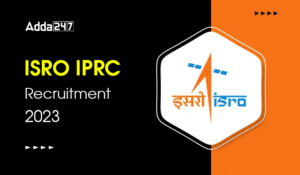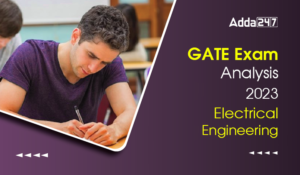Engineering Jobs   »   Mechincal Engineering quizs   »   UPPSC Lecturer Recruitment 2021, UPPSC Lecturer...

# UPPSC Lec’21 ME: Daily Practices Quiz. 08-Dec-2021

Quiz: Mechanical Engineering
Exam: UPPSC Lecturer
Topic: IC Engine

Each question carries 3 marks
Negative marking: 1 mark
Time: 8 Minutes

Q1. The flammable fuels have the flash point at the temperature of
(a) More than the 37.8ºC
(b) Less than 37.8ºC
(c) No temperature limits
(d) Only pressure dependency

Q2. In a 4–cylinder, 4–stroke Diesel engine operating at 1200 rpm, the duration of fuel injection is 20° . The time in seconds during which fuel is injected would be
(a) 1/360 secs
(b) 1/180 secs
(c) 1/720 secs
(d) 1/1440 secs

Q3. In S.I. engine, the throttle valve of carburetor controls the quantity of
(a) Fuel
(b) Air
(c) Fuel and air mixture
(d) Lubricating oil

Q4. Which of the following number decide the ignition quality of diesel fuel?
(a) Octane number
(b) Cetane number
(c) Carbon percentage
(d) Calorific value

Q5. 1 kg of carbon produces following weight of 〖CO〗_2 in flue gases
(a) 44 kg
(b) 22 kg
(c) 11 kg
(d) 11/3 kg

Q6. The volume of charge that can be available in an engine after suction, if the clearance volume is 20 cc and swept volume is 400 cc. Consider mechanical efficiency and volumetric efficiency of engine as 90% and 80% respectively.
(a) 288 cc
(b) 270 cc
(c) 256 cc
(d) 320 cc

Solutions

S1. Ans. (b)
Sol. Flammable fuels have the flash point at the temperature of
below 37.8ºC i.e., 100ºF

S2. Ans. (a)
Sol. Let, the angle turned by crank during fuel injection is θ
N=1200 rpm
So,
ω=2πN/60=(2×π×1200)/60=40π
We know that,
ω=θ/t
t=(π/9)/40π=1/360 sec

S3. Ans. (c)
Sol. In S.I. engine, the throttle valve of carburetor controls the quantity of fuel and air mixture.

S4. Ans. (b)
Sol. Cetane number decide the ignition quality of diesel fuel

S5. Ans. (d)
The chemical equation is
C + O_2 = 〖CO〗_2
1mol+1mol=1mole
12 kg+32kg=44kg
1kg+8/3 kg=11/3 kg
It means that 1 kg of carbon requires 8/3 kg of O_2 for its complete combustion and produce 11/3 kg of 〖CO〗_2

S6. Ans (d)
Sol. Given,
Swept volume (V_s)=400 cc
Clearance volume (V_c)=20 cc
η_m=90%
η_v=80%
η_v=(Actual volume of intake charge)/(Swept volume)=(Actual volume of intake charge)/400=0.8
Actual volume of intake charge=320 cc

Sharing is caring!

•GATE 2023 Admit Card Out, Download Link ...
•GATE Exam Analysis 2023 Computer Science...
•GATE 2023 Memory Based Question Paper of...
•ISRO IPRC Recruitment 2023 Notification ...
•GATE Exam Analysis 2023 Mechanical Engin...
•GATE Exam Analysis 2023 Electrical Engin...
•NIT Warangal Recruitment 2023 Notificati...